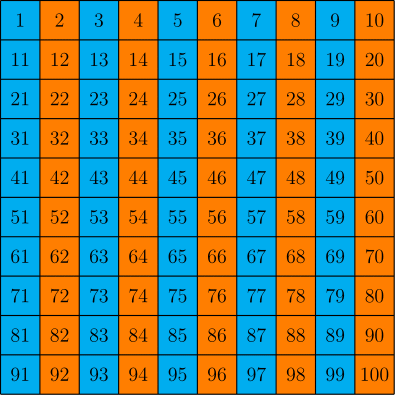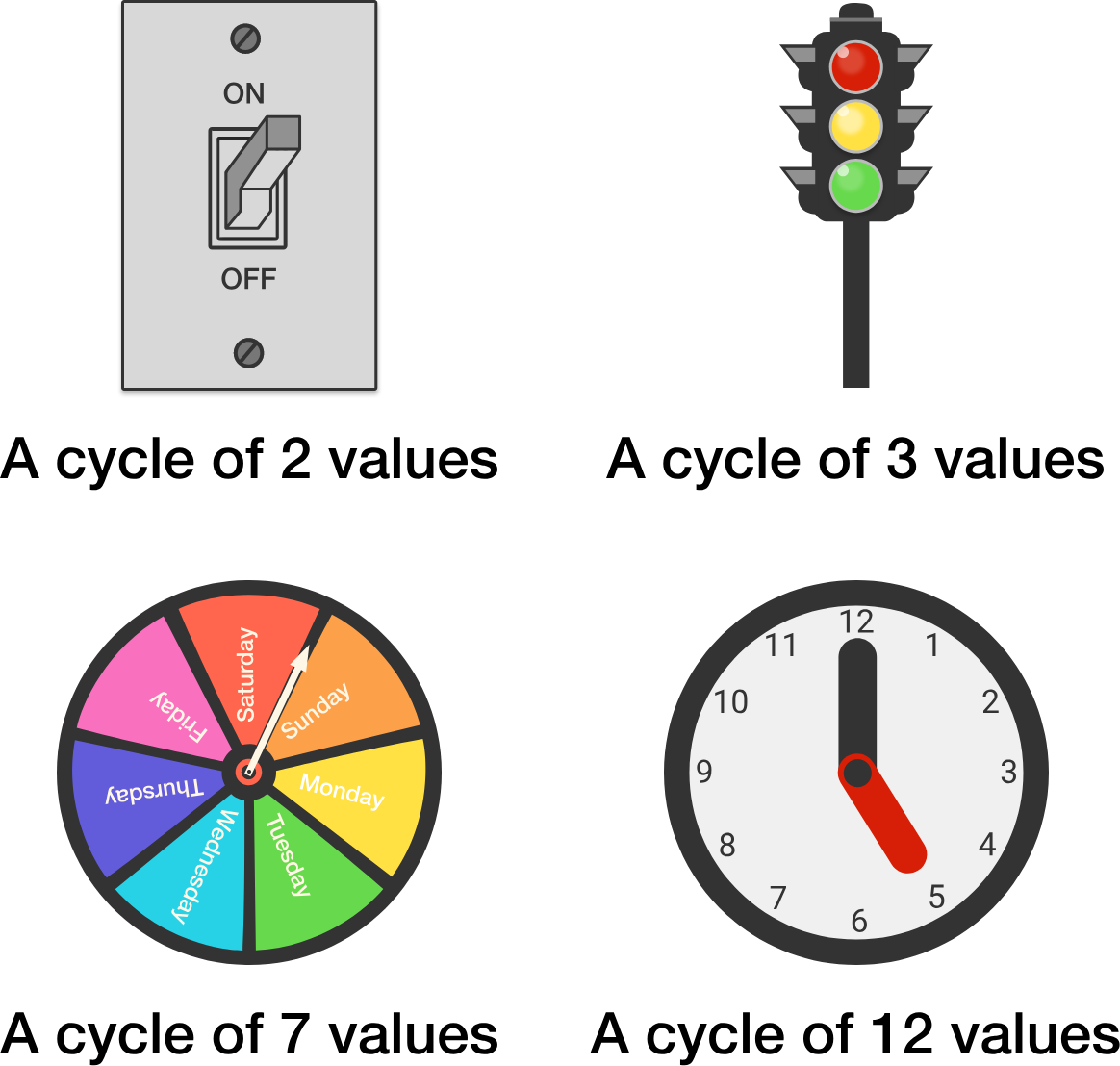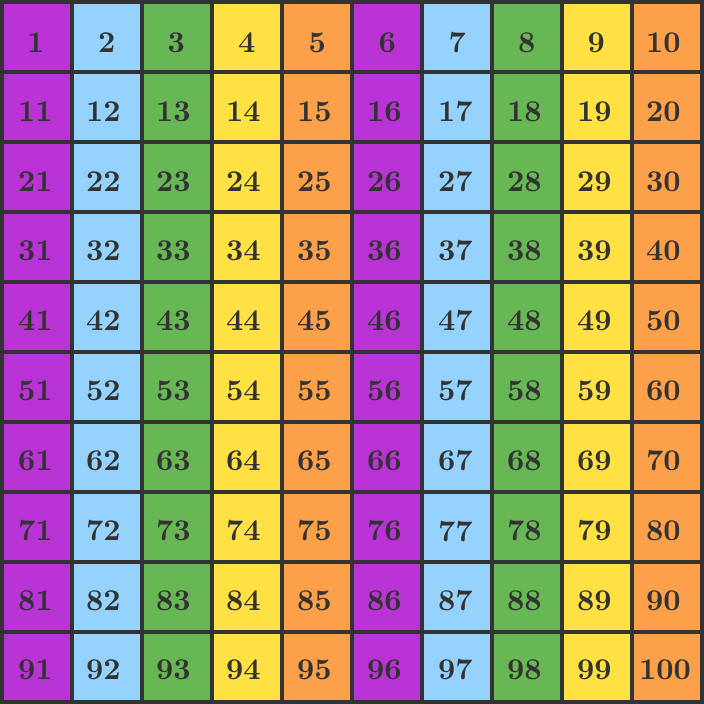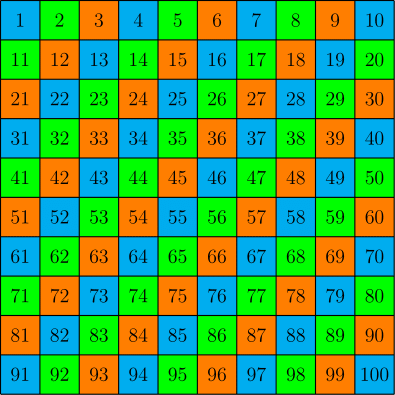### Number TheoryIf all integers are colored in the same pattern as the numbers in this grid, what color is $1234?$

# Rainbow Cycles

Modular arithmetic is the part of number theory used to study cyclical systems: systems where taking enough steps “forward” brings you back to where you started. In this quiz, we'll focus exclusively on abstract coloring patterns so that we can find some general shortcuts for working with these systems.

In the modular arithmetic units later in this course, we'll expand these theories and more into shortcuts that can be applied to many real-life modular systems:# Rainbow CyclesIf you multiply a blue number by an orange number, what color will the product be?

Note: Assume that all integers are colored according to the same pattern as the numbers in the grid.

# Rainbow CyclesIf you add a blue number $($like $2$ or $7)$ to a yellow number $($like $4$ or $9),$ what color will the sum be?

Note: Assume that all integers are colored according to the same pattern as the numbers in the grid.

# Rainbow CyclesIf you multiply a green number by a yellow number, what color will the product be?

Note: Assume that all integers are colored according to the same pattern as the numbers in the grid.

# Rainbow CyclesWhat color is $8 \times 17 ?$

Note: Assume that all integers are colored according to the same pattern (blue, then green, then orange) as the numbers in the grid.

×